# Baskakov operators

(diff) ← Older revision | Latest revision (diff) | Newer revision → (diff)
Jump to: navigation, search

V.A. Baskakov [a2] introduced a sequence of linear positive operators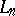with weights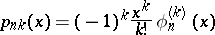by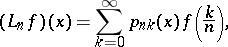(a1)

where,,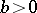, for all functionson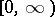for which the series converges. Here,is a sequence of functions defined on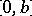having the following properties for every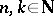,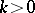:

i)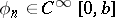;

ii)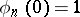;

iii)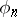is completely monotone, i.e.,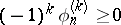;

iv) there exists an integersuch that,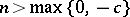.

Baskakov studied convergence theorems of bounded continuous functions for the operators (a1). For saturation classes for continuous functions with compact support, see [a8]. For a result concerning bounded continuous functions, see [a3].

In his work on Baskakov operators, C.P. May [a6] took conditions slightly different from those mentioned above and showed that the local inverse and saturation theorems hold for functions with growth less thanfor some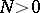. Bernstein polynomials and Szász–Mirakian operators are the particular cases of Baskakov operators considered by May.

S.P. Singh [a7] studied simultaneous approximation, using another modification of the conditions in the original definition of Baskakov operators. However, it was shown that his result is not correct (cf., e.g., [a1], Remarks).

Motivated by the Durrmeyer integral modification of the Bernstein polynomials, M. Heilmann [a4] modified the Baskakov operators in a similar manner by replacing the discrete values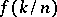in (a1) by an integral over the weighted function, namely,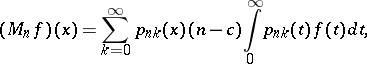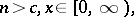whereis a function onfor which the right-hand side is defined. He studied global direct and inverse-approximation theorems for these operators.

Subsequently, a global direct result for simultaneous approximation in the-metric in terms of the second-order Ditzian–Totik modulus of smoothness was proved, see [a5]. For local direct results for simultaneous approximation of functions with polynomial growth, see [a5].